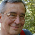## Monday, August 5, 2013

### If you write “the equation,” state the equation (2)

Last year I published a post about people who like to write about “the equation” but never state the equation they’re presumably writing about. Here are four more examples, from a few minutes of Googling:
The web site of a research company called “Equation Research” does not appear to state any equations. (Source)

A web site called “Lori-in-the-equation” does not state the equation, so far as I can see. At one point, Lori mentions “the center of the equation.” At another point Lori suggests that she has left it up to the reader to “define what being in your equation really means.” The web site appears to be about women losing weight. (Source)

A blog called “The Equation: A blog on independent science + practical solutions,” purportedly written by scientists, does not appear to state the equation. As of the time (8/3/2013 1:02 PM EDST) I looked at the blog, most of the recent posts seemed to be about politics, not science. (Source)

A newspaper article with “Critical Latino Vote has Changed the Equation” in the headline does not state what the equation was or what the “critical Latino vote” changed it to. (Source)
The Takeaway: Writing about an equation without stating the equation is flimflam. Write like an honest, intelligent grown-up: If you mean situation, write “situation,” not “equation.” If you mean rules, write “rules,” not “equation.” Whatever you mean, write the word for it; don’t write “equation.” If you don’t know what an equation is, don’t sabotage your credibility by using the word equation.

Update, 8/9/2013 8:47 AM: I removed an example; a reader had pointed out that the equation had indeed been stated.

See disclaimer.

#### 2 comments:

1.Perhaps choose another example? As it is there:

http://procrastinus.com/procrastination-theories/

And here:

http://en.wikipedia.org/wiki/Temporal_motivation_theory

Indeed, it is an equation, and a popular one at that:

http://commonsenseatheism.com/wp-content/uploads/2011/02/Steel-Konig-Integrating-Theories-of-Motivation.pdf

2.Julie horne,

Thank you. I had overlooked those citations.